# Joint Time-Frequency Scattering explanation?

How does JTFS work, intuitively? What are its motivations, and how's it differ from the Wavelet (time) Scattering transform? Can it be visualized?

JTFS is an extension of Wavelet Scattering that exploits time-frequency structure, adding sensitivity to frequency-dependent time shifts, invariance to frequency transposition, and stability against frequency and time-frequency warping.

Core results and intuition are provided in this answer, advanced details in another. Python implementation no longer available anywhere, because Kymatio scrapped my work.

### Joint Time-Frequency Scattering

JTFS remains identical to time scattering up to second order (minus averaging), where it introduces a significant difference; Ref 1:

time scattering has a tree structure. That is, each node only has one parent. In contrast, a general convolution network sums contributions from multiple nodes in a layer to produce a node in next layer.

JTFS breaks the tree structure by convolving along frequency, exploiting the joint time-frequency geometry. In detail:

\begin{align} S_{(J, J_{fr})}^{{(0)}} x(t) &= x \star \phi_T(t), \\ S_{(J, J_{fr})}^{{(1)}} x(t, \lambda) &= |x \star \psi_\lambda^{{(1)}}| \star \phi_T, \\ S_{(J, J_{fr})}^{{(2)}} x(t, \lambda, \mu, l, s) &= ||x \star \psi_\lambda^{{(1)}}| \star \Psi_{{\mu, l, s}}| \star \Phi_{(T, F)}. \end{align}

We hence convolve $$U_1$$ (|CWT|) with joint wavelets. Full description in other answer.

### FDTS

JTFS's primary addition is sensitivity to Frequency-Dependent Time Shifts - transformations of form

$$X(\lambda, t) \rightarrow X(\lambda, t - \tau(\lambda))$$

where $$X=\text{CWT}(x)$$. Time scattering is insensitive since it's invariant to time shifts.

Illustrating, take windowed pure sines and shift them more with greater frequency:Left is the signal (zoomed), right its scalogram, first on bottom is time scattering, second is JTFS (spinned coefficients only), latter two with $$T=\text{signal duration}$$. Time scattering doesn't visibly change at all, while a fair portion of JTFS does. The relative distances, $$||\Phi_0 - \Phi_1|| / ||\Phi_0||$$, are:

TS      = 0.0012
JTFS    = 0.2056
JTFS/TS = 168.1


The ratio lessens with lower $$T$$, and stays high when including all JTFS coefficients. Ratio lessens with latter since only spinned coefficients are FDTS-sensitive.

### Frequency transposition invariance

"Frequency transposition" here means a shift in log-frequency, in identical time-frequency geometric sense to a shift in time: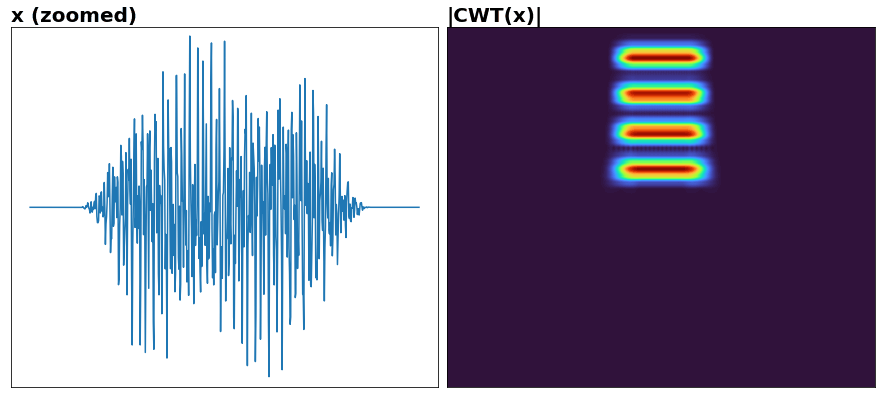All time-shift invariance apply analogously to log-frequency shifts. Invariance is also imposed to linear shifts but amount of invariance is determined in a nonlinear manner.

### Joint filterbank

Is product of temporal and frequential; example of latter:Joint (2D), where empty spots are what's omitted in calculation per symmetry (and would fill symmetrically), and (spatially) small filters dropped for clarity: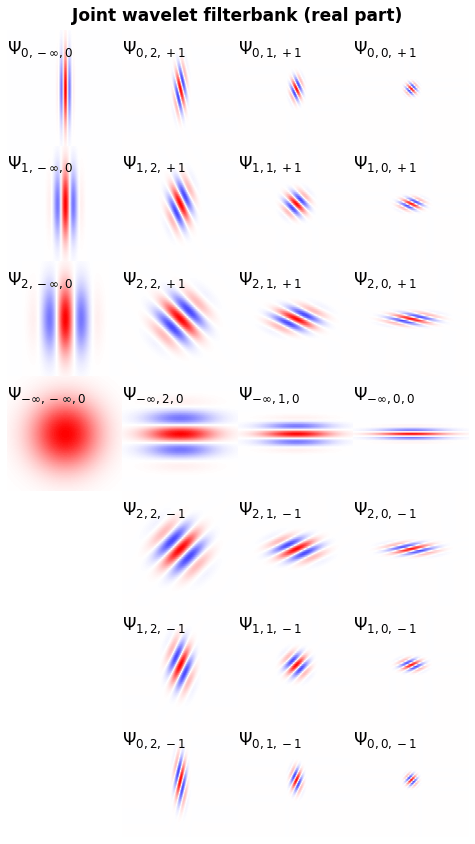### Example: exponential chirp

An echirp can be formed by shifting a delta (unit impulse), by a constant in time per a constant in log-frequency. Despite being remarkably different, time scattering cannot distinguish them.

Let's see how JTFS fares:Recall, a JTFS coefficient is output from a 2D convolution; we visualize with each such "joint slice" individually: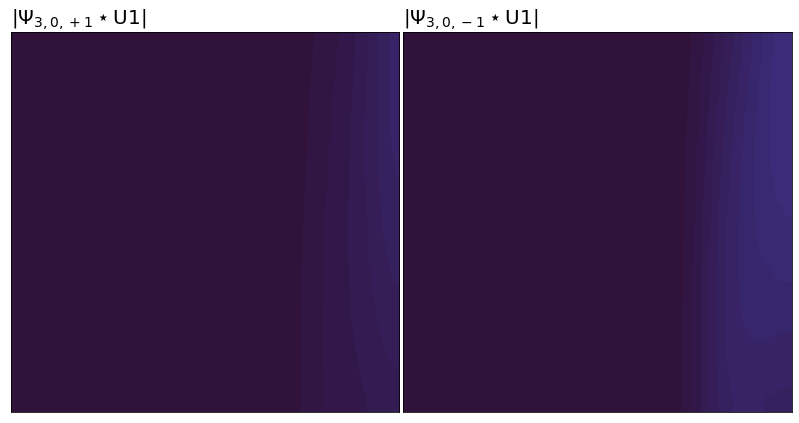Taking ratio of spinned coefficients' energies:

E_down / E_up = 73.6


This spin assymetry illustrates FDTS discriminability. The ratio can be driven far greater with proper discretization, but not up to the theoretical ideal of infinity per finiteness limitations.

Focusing on down spin and lowpass, and changing the filterbank to produce fewer coefficients for clarity, let's show wavelets and the coefficients they produce:The frequencies per slice are arranged low-to-high going top-to-bottom, as are the slices, opposite of the first GIF.

• The joint lowpass pair, phi_t * phi_f, hoards the most energy, in accords with scattering Energy Analysis.
• The largest spinned pair is one whose orientation matches the chirp's in time-logfrequency (scalogram). A GIF of sweeping fmax from N/2 to fmin, then fixing fmax and sweeping fmin up to N/2 (reverse chirp), would show different spinned pairs peaking, and switching down to up at chirp reversal.

### True JTFS structure

Is 4D: (time, freq, log-freq, log-quef) = (t, xi1, xi2, xi1_fr). Taking the same echirp with approximately same filterbank, we can visualize as 3D slices unfolded over time: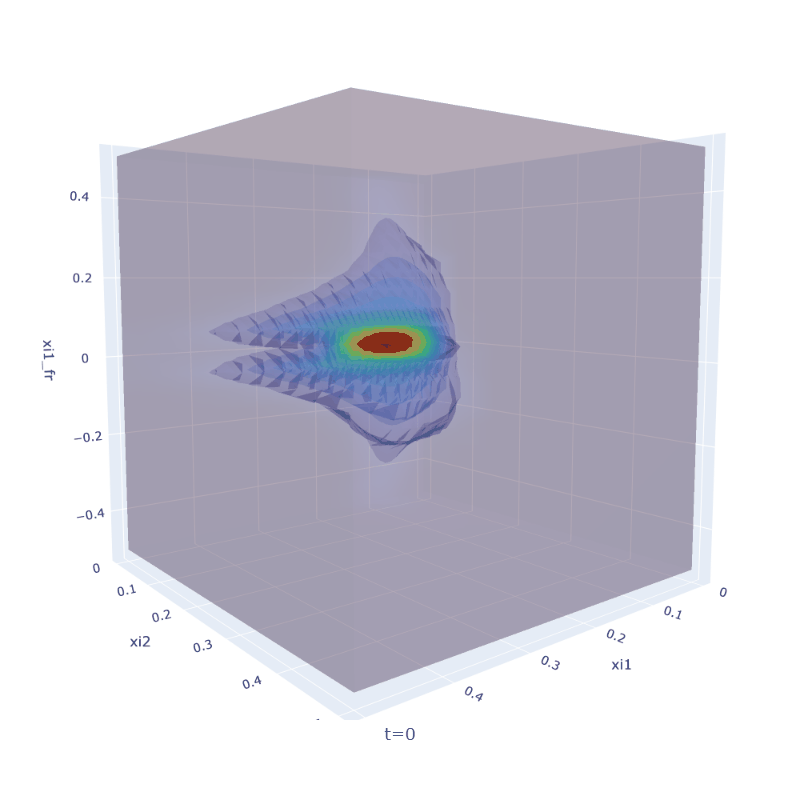Energy shifts toward greater xi1 (frequency) over time, as expected for up chirp. Note the asymmetry of spins, and DC portion consistently dominating the overall representation. Former is more obvious by showing spinned only: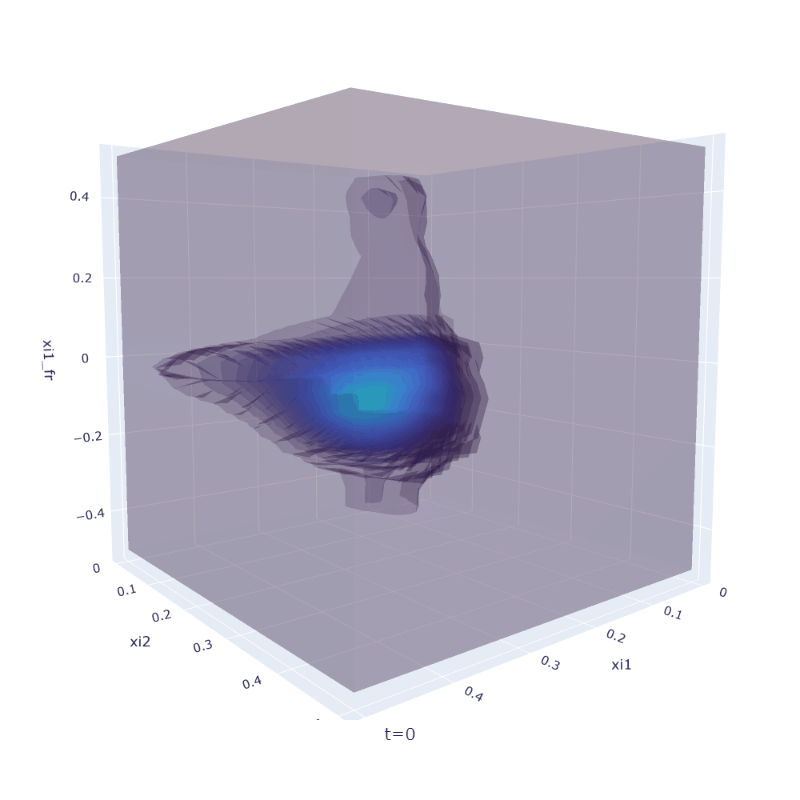Note all examples, existing and to follow, obeying the scattering energy flow: xi2 < xi1 $$\Leftrightarrow f_\text{modulator} < f_\text{carrier}$$.

### EEG: seizures, ictal period

10 minutes, one channel, $$T=2.6\ \text{sec}$$, spinned only: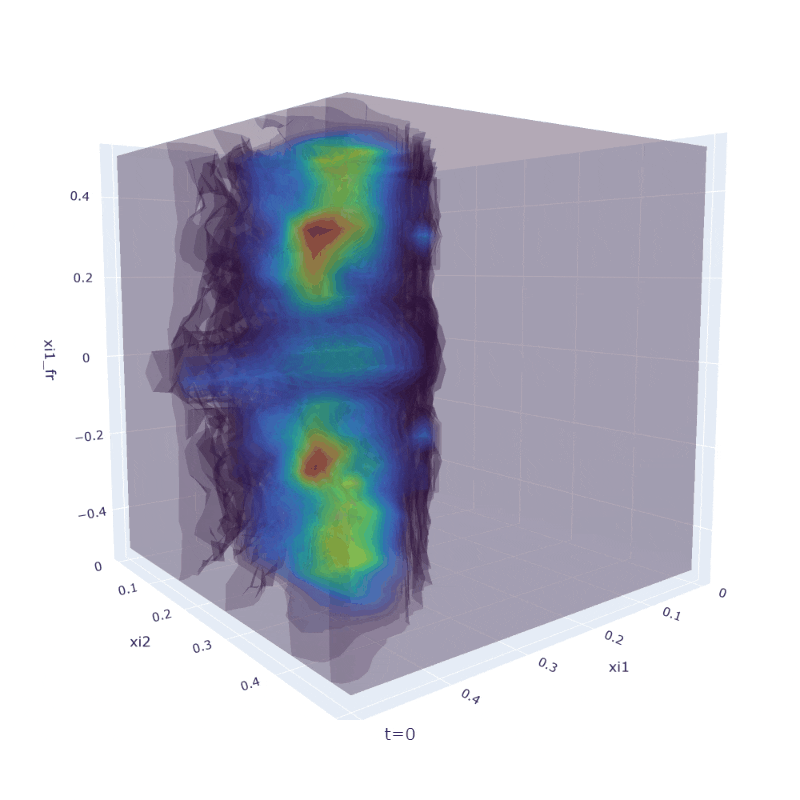### Audio: trumpet

y, sr = librosa.load(librosa.ex('trumpet')); sounddevice.play(y, sr) ~same relative configuration as in EEG: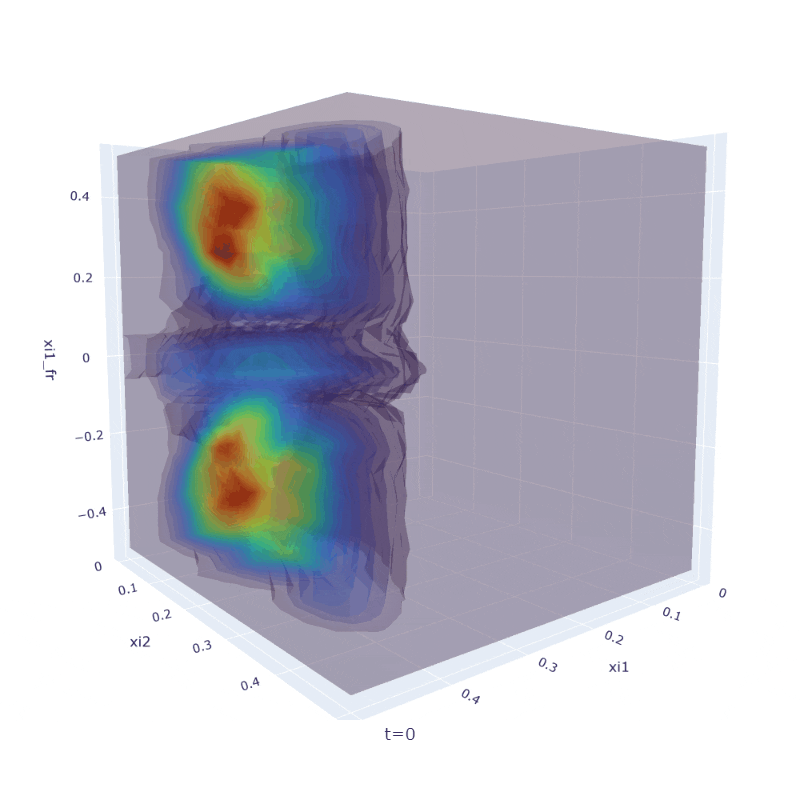### Bioplausibility

JTFS shares bioplausibility with STRF (Spectro-Temporal Receptive Fields), where close correspon-dence with auditory responses of animals and humans is shown by neurophysiological and behavioral evidence (Ref 2, D. Klein, et al, F. Theunissen, et al). Methods include comparing direct measurements of auditory cortex responses with the model's coefficients to a range of stimuli, and correlating perceptual recognition of human listeners of musical sounds with nearest neighbor search directly on coefficients.

T. Chi, et al estimate $$Q_2 \approx 2$$ (second-order temporal filterbank's $$Q$$) in humans.

### Applications

JTFS is applicable anywhere time scattering is (see "Applications"), and particularly anywhere FDTS and frequency transposition invariance are beneficial:

• Audio texture synthesis
• Phone segment classification
• Musical instrument classification
• Acoustic scene classification

Quoting Ref 1:

improvement [in instrument classification] likely stems from the fact that musical instruments carry important discriminative information in the temporal evolution of their spectral envelopes as well as frequency modulation structures, both of which are captured by joint decompositions in time and log-frequency

The timbre of a musical instrument is essentially determined by its shape and materials. Both remain constant during a musical performance. Therefore, musical instruments may be modeled as dynamical systems with constant parameters. The task of musical instrument classification is to retrieve these parameters while remaining invariant to changes in pitch, intensity, and expressive technique induced by the performer.

a signal with many transients, such as a jackhammer in a street scene, and misaligning its subbands yields a completely different sound

Note there need not be structures explicitly shifted in time depending on frequency; JTFS doesn't know, nor cares, whether this occurred. All that's needed is for time-frequency ridges to vary, or begin and end, which is true for anything that isn't an everlasting sine; JTFS spins will uniquely extract these features which may lack a familiar interpretation but nonetheless be useful.

### Libraries

I have implemented JTFS in Python, currently not available anywhere.

MATLAB implementations are available at scattering.m and ScatNet. With disclaimer that I'm the author, the Kymatio implementation is the most flexible and validated of any I know.